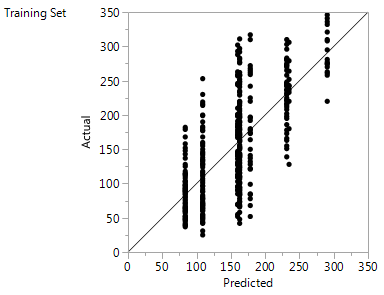For the latest version of JMP Help, visit JMP.com/help.

Publication date: 11/29/2021

## Actual by Predicted Plot

For continuous responses, the Actual by Predicted plot is the typical plot of the actual response versus the predicted response. When you fit a Decision Tree, all observations in a leaf have the same predicted value. If there are n leaves, then the Actual by Predicted plot shows at most n distinct predicted values. The actual values form a scatter of points around each leaf mean on n vertical lines.

The diagonal line is the Y = X line. For a perfect fit, all the points would be on this diagonal. When validation is used, plots are shown for both the training and the validation sets (Figure 4.16).

Figure 4.16 Actual by Predicted Plots for a Continuous ResponseWant more information? Have questions? Get answers in the JMP User Community (community.jmp.com).